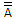# Digital Electronics - Boolean Algebra and Logic Simplification

Exercise : Boolean Algebra and Logic Simplification - True or False
1.
A variable is a symbol used to represent a logical quantity that can have a value of 1 or 0.
True
False
Explanation:
No answer description is available. Let's discuss.

2.
The OR function is Boolean multiplication and the AND function is Boolean addition.
True
False
Explanation:
No answer description is available. Let's discuss.

3.
In Boolean algebra, A + 1 = 1.
True
False
Explanation:
No answer description is available. Let's discuss.

4.
The product-of-sums (POS) is basically the ORing of ANDed terms.
True
False
In Boolean algebra,= A.